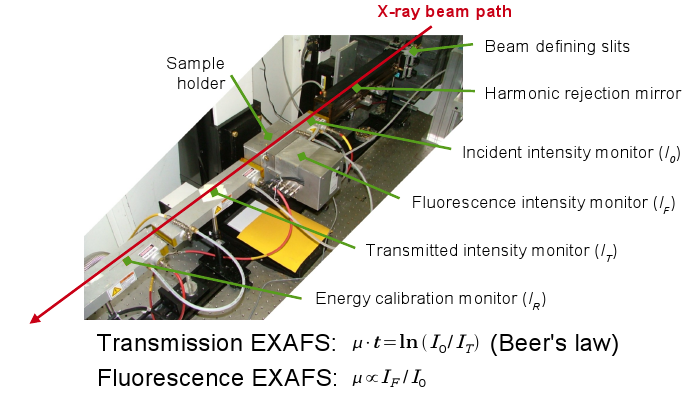# Energy Calibration Standards

## What purpose does the calibration standard serve?

Most EXAFS beamlines use double crystal monochromators. A DCM consists of two parallel crystal planes. These planes are rotated to present the crystals to the incoming white beam at specified angles. The angle is chosen such that the crystals meet the Bragg condition for the desired energy. The energy dependence of the EXAFS signal thus is obtained by scanning the DCM through an angular range corresponding to the energy range of the experiment.

In an ideal EXAFS experiment, the DCM would return to the exact starting angle of each scan. In practice, there are a number of reasons that a DCM might not return to the exact right position. For example, if a DCM is driven by a stepper motor, steps might be lost – either in the signal chain or in the mechanical coupling. Or a DCM might change temperature between scans such that the lattice constant of the crystal changes. In either case, the actual energy range measured in successive scans might be slightly different.

In order to interpret scans measured under these conditions, it is essential that the data can be aligned in energy. That is, we need a way to post-process the data to correct for any variations in the behavior of the DCM. (When building a beamline from scratch, it is a good idea to use an encoder on the Bragg angle axis and use encoder position to determine your energy. In this way, energy calibration post-processing is often not necessary.)

The most common solution is to measure a reference standard in parallel with the measurement on your sample. Measured in parallel, it is guaranteed that the standard and the sample share an energy axis in the resulting data file. If the same standard is measured with each different sample, then we have a way of aligning our data. If we align the reference spectra and use the same shift in energy to correct the associated sample spectra, then we know that our sample spectra are aligned precisely. This has been integrated in most data analysis software packages.

## How is the calibration standard used?

### Transmission reference chamberFig 1. Setup of a typical XAFS experiment at a rather old-fashioned XAFS beamline

The most common method of measuring a calibration reference is to place a calibration standard after the ion chamber used to measure the transmission signal. This is followed by another ion chamber, often called the reference chamber. In Figure 1, you can see that two ion chambers are placed after the sample.

The reference signal, then, is a transmission EXAFS measurement using IT to measure the incident intensity and IR to measure the transmitted intensity through the reference sample. The signals from these two detectors are written to the same data file as the rest of the signals measured in the experiment. The reference is measured completely in parallel with the sample and the data from the IR detector is measured on the exact same same energy axis as the data from the sample.

If the same standard is used in every measurement of every sample, then it can be used to align all data to a common energy axis. With the parallel reference measurement, this energy alignment can be performed unambiguously regardless of the quality of the data from the sample and regardless of the valence state of the sample. Thus changes in edge position as a function of valence state can be measured with high precision.

The quality of the data measured on the reference channel is often rather suspect. This cannot be avoided in many situations. If the sample or its containment is highly absorbing, there may not be many photons left by the time they get to the IR chamber. What’s more, the signal on IT is used to measure the intensity incident on the standard. The IT signal, however, is almost certainly changing due to the absorption of the sample itself. The IR signal is, therefore, quite sensitive to the homogeneity and linearity of all other parts of the experiment. It is relatively rare that the reference measurement is of similar quality to a proper EXAFS measurement on that same material. This is not a catastrophe, though, because the only part of the reference signal that matters is the bit around the edge. That is the part that is used to define the energy calibration. Since the edge is the most swiftly varying part of the data, it can be used to good effect for energy calibration even if the reference data are of poor overall quality.